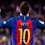# My discussions $1$

Some awesome problems that I loved to solve, Try solving them they are fun...

($1$)

If $a_1,a_2,a_3...............a_{24}$ are such that

$\dfrac{a_2}{a_1}= \dfrac{a_3}{a_2}=............ =\dfrac{a_{24}}{a_{23}}$

and $\displaystyle\sum_{i=1}^{24}$$a_i = 2$

$\displaystyle\sum_{i=1}^{24}$$\dfrac{1}{a_i} = 1$

Find $\displaystyle\prod_{i=1}^{24}$$a_i$

($2$)

For an acute-angled $\triangle{ABC}$ ] $0,\pi/2$] , proove that (without using AM-GM)

$\sin(A). \sin(B). \sin(C) \leq \dfrac{3\sqrt3}{8}$

($3$)

Find the largest constant $k$ such that

$\dfrac{kabc}{a+b+c} \leq (a+b) ^2+(a+b+4c)^2$

($4$)

Find the real roots of the equation

$x^2+2ax+\dfrac{1}{16} = - a+\sqrt{a^2+x-\dfrac{1}{16}}$

($5$)

Proove that

$1 <\dfrac{1}{1001} + \dfrac{1}{1002} +............ +\dfrac{1}{3001} < \dfrac{4}{3}$

Hope you will enjoy them!!Note by Parth Lohomi
6 years, 5 months ago

This discussion board is a place to discuss our Daily Challenges and the math and science related to those challenges. Explanations are more than just a solution — they should explain the steps and thinking strategies that you used to obtain the solution. Comments should further the discussion of math and science.

When posting on Brilliant:

• Use the emojis to react to an explanation, whether you're congratulating a job well done , or just really confused .
• Ask specific questions about the challenge or the steps in somebody's explanation. Well-posed questions can add a lot to the discussion, but posting "I don't understand!" doesn't help anyone.
• Try to contribute something new to the discussion, whether it is an extension, generalization or other idea related to the challenge.

MarkdownAppears as
*italics* or _italics_ italics
**bold** or __bold__ bold
- bulleted- list
• bulleted
• list
1. numbered2. list
1. numbered
2. list
Note: you must add a full line of space before and after lists for them to show up correctly
paragraph 1paragraph 2

paragraph 1

paragraph 2

[example link](https://brilliant.org)example link
> This is a quote
This is a quote
    # I indented these lines
# 4 spaces, and now they show
# up as a code block.

print "hello world"
# I indented these lines
# 4 spaces, and now they show
# up as a code block.

print "hello world"
MathAppears as
Remember to wrap math in $$ ... $$ or $ ... $ to ensure proper formatting.
2 \times 3 $2 \times 3$
2^{34} $2^{34}$
a_{i-1} $a_{i-1}$
\frac{2}{3} $\frac{2}{3}$
\sqrt{2} $\sqrt{2}$
\sum_{i=1}^3 $\sum_{i=1}^3$
\sin \theta $\sin \theta$
\boxed{123} $\boxed{123}$

Sort by:

Solution for( 2)

F(x)=$\log(\sin(x))$

F'(x)=$\cot(x)$

F"(x)= -$cosec(x)$

Apply jensens inequality

$F(\dfrac{A+B+C}{3}) \geq \dfrac{F(A)+F(B) +F(C)}{3}$

log(sin($\pi/3$) $\geq \dfrac{log(sin(A)) +log(sin(B))+log(sin(C))}{3}$

3 log($\dfrac{\sqrt{3}}{2}$) $\geq \log(\sin A+\sin B+\sin C)$

Thus

$\boxed{(\sin A. \sin B. \sin C) \leq \dfrac{3\sqrt3}{8}}$

- 6 years, 5 months ago

Rather directly apply on $\sin x$.

- 6 years, 5 months ago

(2)

$A,B,C<\pi \Rightarrow\sin A, \sin B, \sin C >0$

Now consider $\sin A , \sin B , \sin C$.

We know that $GM≤AM$, and hence the maximum value will occur only when $GM=AM$, i.e. the terms are equal.

$\therefore \sin A = \sin B = \sin C \Rightarrow A=B=C$

As $A+B+C=\pi$, we have $A=B=C=\frac{\pi}{3}$

Therefore, the maximum value of $\sin A \sin B \sin C$ occurs at $A=B=C=\frac{\pi}{3}$.

$\therefore \sqrt{\sin A \sin B \sin C} \le \frac{\sin A + \sin B + \sin C}{3}$

$\sqrt{\sin A \sin B \sin C} \le \sin\frac{\pi}{3}$

$\sqrt{\sin A \sin B \sin C} \le \frac{\sqrt{3}}{2}$

$\boxed{\sin A \sin B \sin C \le \frac{3\sqrt{3}}{8}}$

- 6 years, 5 months ago

Sorry I forgot to mention without AM-GM

- 6 years, 5 months ago

Oh :P

- 6 years, 5 months ago

5) Let $1001 \leq k \leq 3001$

A.M - H.M inequality -

$\displaystyle \left(\sum_{k=1001}^{3001}k\right)\left( \sum_{k=1001}^{3001} \dfrac{1}{k}\right) > (2001)^2$

$\displaystyle \sum_{k=1001}^{3001} k = (2001)^2$

Thus, $\displaystyle \sum_{k=1001}^{3001} \dfrac{1}{k} > 1$

$M = \displaystyle \sum_{k=1001}^{3001} \dfrac{1}{k} < \dfrac{500}{1000} + \dfrac{500}{1500} + \dfrac{500}{2000} + \dfrac{1}{3001}$ ( grouped 500 terms simultaneously)

$< \dfrac{1}{2} + \dfrac{1}{3} + \dfrac{1}{4} + \dfrac{1}{5} + \dfrac{1}{3000} = \dfrac{3851}{3000} < \dfrac{4}{3}$

$\boxed{1 < \dfrac{1}{1001} + .... + \dfrac{1}{3001} < \dfrac{4}{3}}$

- 6 years, 5 months ago

Good solution @megh choksi

- 6 years, 5 months ago

I think the last question should be -

$1 < \dfrac{1}{1001} + ...... + \dfrac{1}{3001} < \dfrac{4}{3}$

- 6 years, 5 months ago

Oops corrected! !

- 6 years, 5 months ago

4) Good one , we can see RHS is inverse of LHS , thus

$x^2 + 2ax + \dfrac{1}{16} = x$

$x^2 + x(2a - 1) + \dfrac{(2a - 1)^2}{4} = \dfrac{(2a - 1)^2}{4} + \dfrac{1}{16}$

$(x + (\dfrac{2a - 1}{2})^2 = \dfrac{(2a - 1)^2}{4} + \dfrac{1}{16}$

$\implies x = \dfrac{2a - 1}{2} \pm \sqrt{\dfrac{(2a - 1)^2}{4} + \dfrac{1}{16}}$

Real roots depends on a.

From our equation - $x^2 + x(2a - 1) - \dfrac{1}{16}$ , we can say for real roots discriminant should be greater than or equal to zero thus

$(2a - 1)^2 - \dfrac{1}{16} \geq 0$

$\implies a \leq \dfrac{1}{4} ~or~ a \geq \dfrac{3}{4}$

- 6 years, 5 months ago